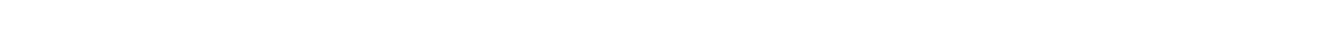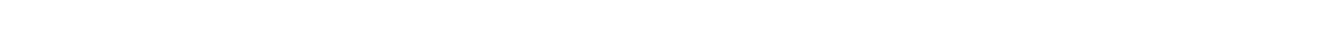# 2.3.3 The Weak Interaction

### The Weak Interaction

• The weak interaction is responsible for the radioactive decay of atoms
• The exchange particle that carries this force is the W, W+ or Z0 boson
• The type of exchange particle depends on the type of interaction

#### β decay

• β and β+ decay are examples of the weak interaction in action
• In β decay, a neutron turns into a proton emitting an electron and an anti-electron neutrino• The W boson is the exchange particle in this interactionFeynman diagram showing beta minus decay. The W boson is the exchange particle

• In β+ decay, a proton turns into a neutron emitting a positron and an electron neutrino• The W+ boson is the exchange particle in this interactionFeynman diagram showing beta plus decay. The W+ boson is the exchange particle

#### Electron Capture & Electron–Proton Collisions

• Electrons and protons are attracted to each other via the electromagnetic interaction
• However, when they interact with each other, it is the weak interaction that facilitates the collision
• Both electron capture and electron-proton collisions have the same decay equation• Electron capture is when an atomic electron is absorbed by a proton in the nucleus resulting in the release of a neutron and an electron neutrino
• This decay is mediated by the W+ boson
• Electron-proton collisions are similar; when an electron collides with a proton, a neutron and an electron neutrino are emitted
• This decay is mediated by the W bosonFeynman diagrams for electron capture and an electron-proton collision. These are equal except for the sign of the W boson

#### Exam Tip

Notice that the sign of the W boson matches that of the beta decay. The W boson is exchanged in beta minus decay and W+ boson is exchanged in beta plus decay. The sign should reflect the total charge of the products (right-hand side) in the equation.### Author: Ashika

Ashika graduated with a first-class Physics degree from Manchester University and, having worked as a software engineer, focused on Physics education, creating engaging content to help students across all levels. Now an experienced GCSE and A Level Physics and Maths tutor, Ashika helps to grow and improve our Physics resources.
CloseClose

### Download all our Revision Notes as PDFs

Try a Free Sample of our revision notes as a printable PDF.

Already a member?